# KNN Vs. K-Means

## Introduction

We’ll be learning about two famous machine learning algorithms, K Nearest Neighbours(KNN) and K-Means.

These two algorithms are often confused due to the letter 'K' in both of them; however, both are different from each other.

KNN is a supervised machine learning algorithm, while on the other hand, K-Means is an unsupervised machine learning algorithm.

## What is KNN?

KNN is a supervised machine learning algorithm that is used for classification problems. Since it is a supervised machine learning algorithm, it uses labeled data to make predictions.

KNN analyzes the 'k' nearest data points and then classifies the new data based on the same.

In detail, to label a new point, the KNN algorithm analyzes the ‘k’ nearest neighbors or ‘k’ nearest data points to the new point. It chooses the label of the new point as the one to which the majority of the ‘k’ nearest neighbors belong to.

## KNN Algorithm

The various steps involved in KNN are as follows:-

→ Choose the value of ‘K’  where ‘K’ refers to the number of nearest neighbors of the new data point to be classified.

→ Now, compute the euclidian distance between the new input (new data point) and all the training data.

Example: Let us assume we have two points, A1(X1, Y1) and B2(X2, Y2). Then the euclidian distance between the two points would be the following:-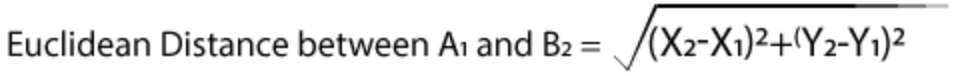→ Sort these distances in ascending order and choose the first ‘K’ minimum distance values. This will give us the ‘K’ nearest neighbors of the new data point.

→ Now, find out the label/class to which all these neighbors belong.

→ Find the majority class these neighbors belong to and assign that particular label to the new input.

→ Finally, return the predicted class of the new data point.

Note: It is essential to choose an appropriate value of ‘K’ to avoid the overfitting of our model.

Now that we’ve discussed the implementation of KNN, we will be moving on to K-Means.

## What is K-Means?

K-Means is an unsupervised machine learning algorithm that is used for clustering problems. Since it is an unsupervised machine learning algorithm, it uses unlabelled data to make predictions.

K-Means is nothing but a clustering technique that analyzes the mean distance of the unlabelled data points and then helps to cluster the same into specific groups.

In detail, KNN divides unlabelled data points into specific clusters/groups of points. As a result, each data point belongs to only one cluster that has similar properties.

## K-Means Algorithm

The various steps involved in K-Means are as follows:-

→ Choose the 'K' value where 'K' refers to the number of clusters or groups.

→ Randomly initialize 'K' centroids as each cluster will have one center. So, for example, if we have 7 clusters, then we would initialize seven centroids.

→ Now, compute the euclidian distance of each current data point to all the cluster centers. Based on this, assign each data point to its nearest cluster. This is known as the 'E- Step.'

Example: Let us assume we have two points, A1(X1, Y1) and B2(X2, Y2). Then the euclidian distance between the two points would be the following:-→ Now, update the cluster center locations by taking the mean of the data points assigned. This is known as the 'M-Step.'

→ Repeat the above two steps until convergence, i.e., until we reach a global optimum where no further optimization is possible.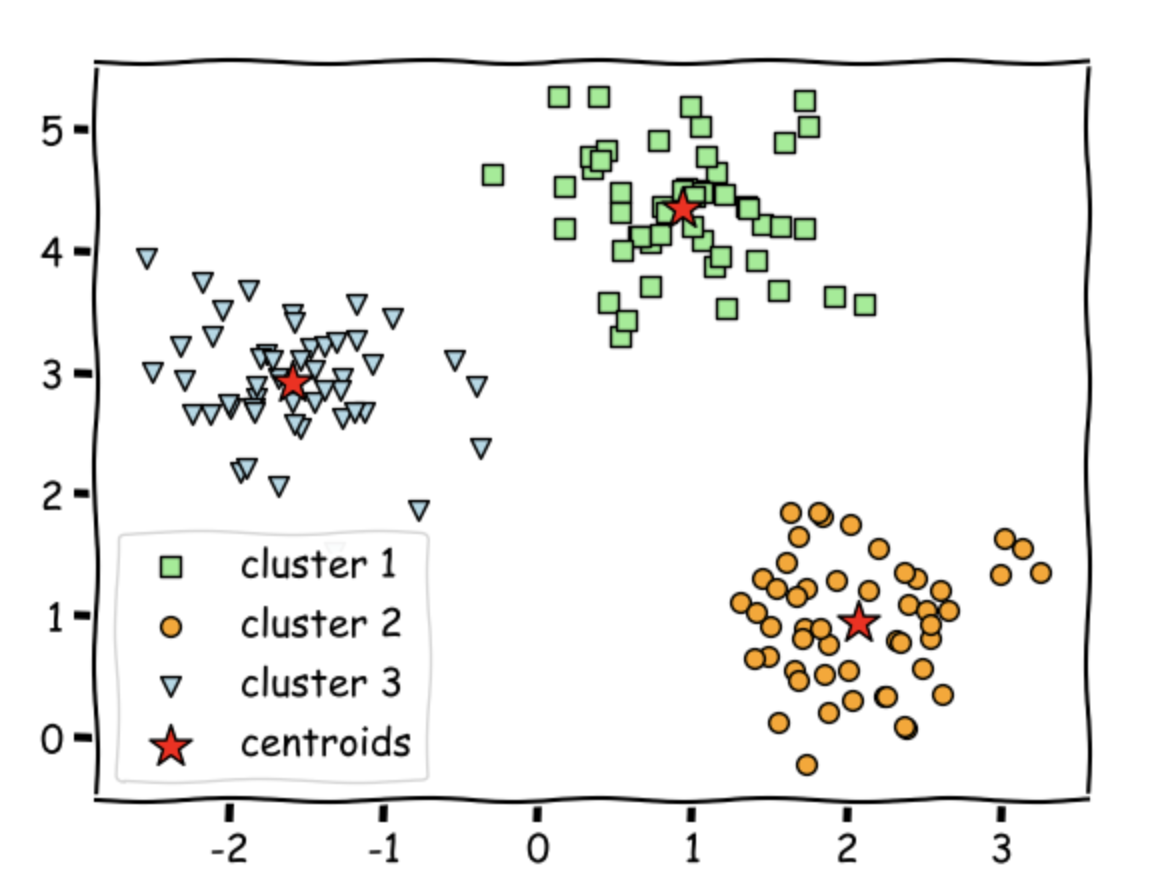## Dataset

We will be using the Iris Dataset for testing both the algorithms - KNN and K-Means.

The Iris Dataset helps predict the Iris flower species based on a few given properties. It consists of 5 features and one target variable.

(i) Id - ID of the flower for differentiating, numerical feature.

(ii) SepalLengthCm - sepal length of the flower, numerical feature.

(iii) SepalWidthCm - sepal width of the flower, numerical feature.

(iv) PetalLengthCm - petal length of the flower, numerical feature.

(v) PetalWidthCm - petal width of the flower, numerical feature.

(vi) Species - iris species , target variable / label.

## Implementation

For simplicity, we would use the already existing sklearn library for KNN and K-Means implementation.

## Importing Necessary Libraries

Firstly, we will load some basic libraries:-

(i) Numpy - for linear algebra.

(ii) Pandas - for data analysis.

(iii) Seaborn - for data visualization.

(iv) Matplotlib - for data visualisation.

(v) KNeighborsClassifier - for using KNN.

(vi) KMeans - for using K-Means.

(vii) classification_report - for generating numerous results.

## Visualization

We visualize the dataset by printing the first ten rows of the data frame. We use the head() function for the same.

Output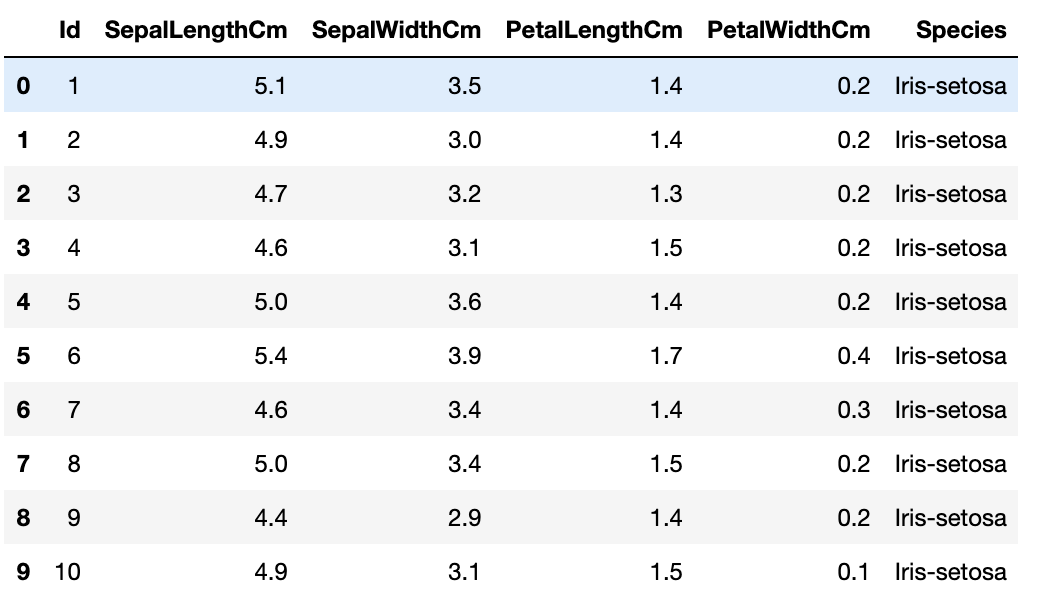Output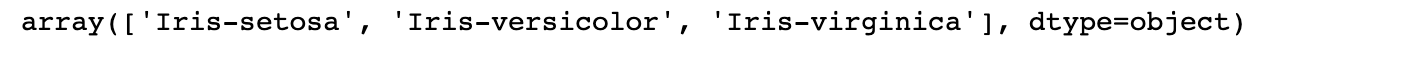We notice that there are three different classes.

Output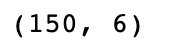The dataset has 150 egs and 6 columns (including one target variable).

Output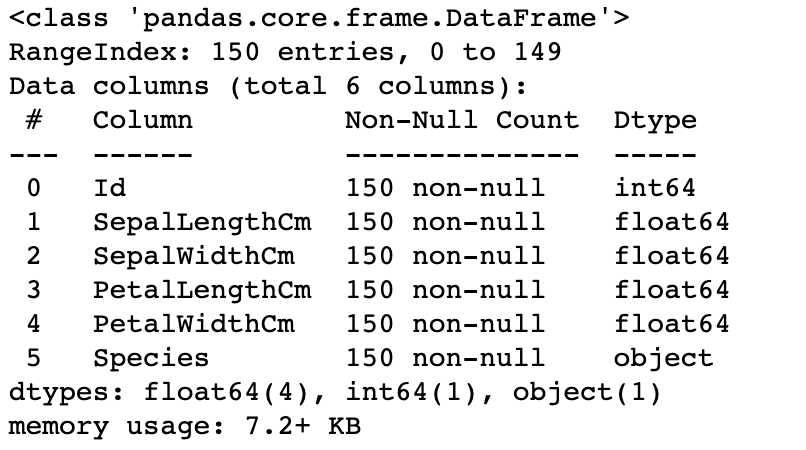Output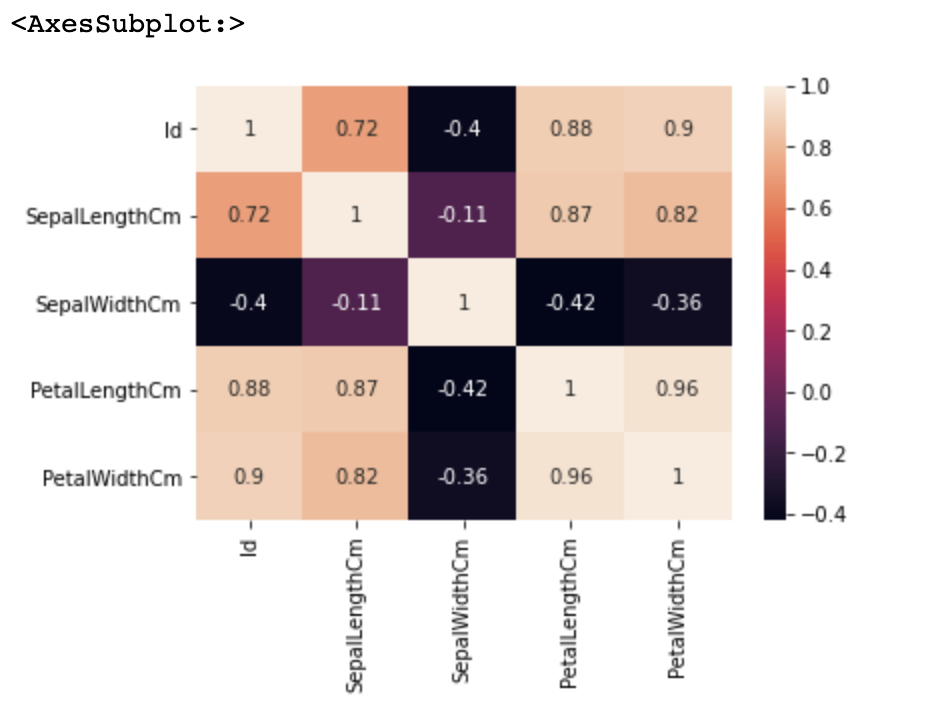## Preprocessing

### Data imputation

Output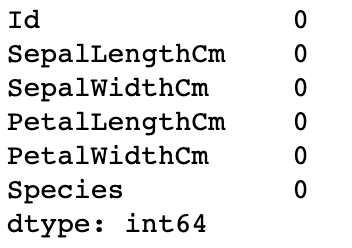We observe that the dataset does not contain any Null values.

### Label Encoding

We perform label encoding for converting the categorical feature ‘Species’ into a numerical one.

Output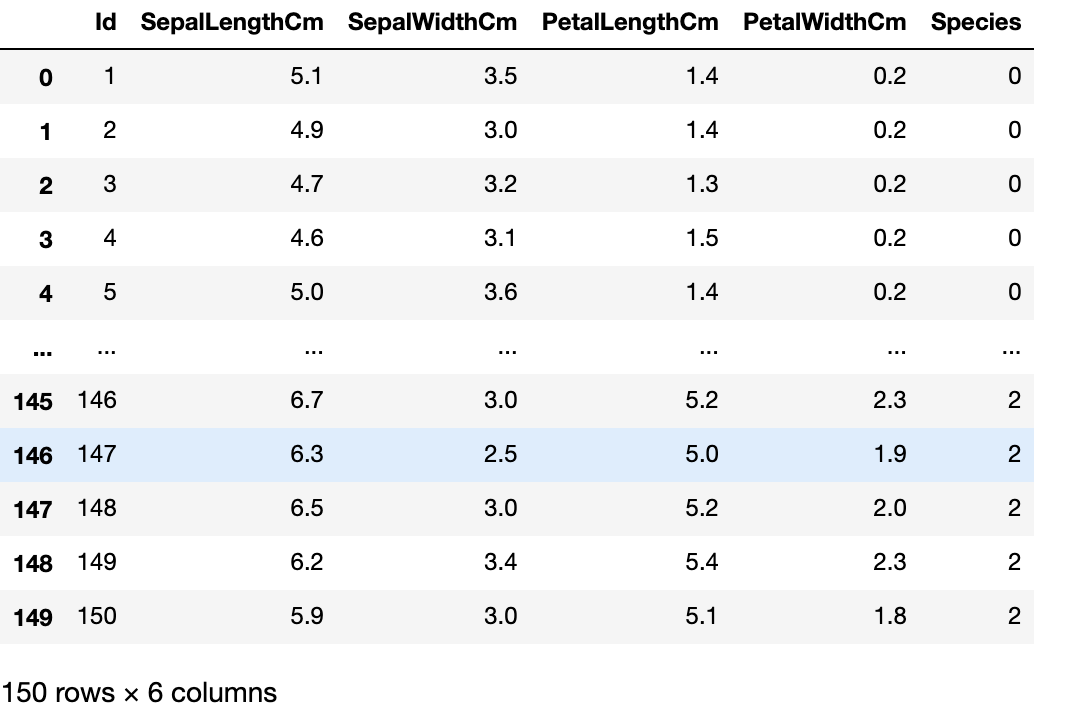Output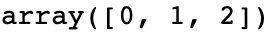As noticeable above, all target values are now numerical.

### Insignificant Features

We drop ‘ID’ as this feature is insignificant.

Output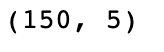Now, we have just 150 examples and 5 columns.

### Train-Test Split

Now, we will divide our data into training data and testing data. We will have a 3:1 train test split.

Output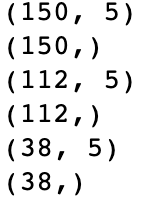## Training

We will build our KNN and KMeans models using the sklearn library and then train them.

### Results

Now, we analyze our models and generate the results.

Output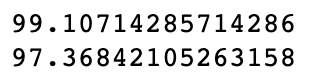We notice that we get good results on both training and testing sets for KNN. The training set gives us a score of 99.10, whereas the testing set gives us a score of 97.36.

Output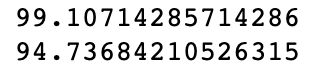We notice that we get good results on both training and testing sets for KMeans too. The training set gives us a score of 99.10, whereas the testing set gives us a score of 94.73.

1. What is the difference between KNN and KMeans?
The main difference is that KNN is a supervised machine learning algorithm used for classification, whereas KMeans is an unsupervised machine learning algorithm used for clustering.

On the positive side, KNN is very easy to implement, whereas, on the negative side, KNN does not work too well with a large dataset.

An advantage of KMeans is that it is computationally very fast. A disadvantage of the same is that it does not work too well with clusters of different sizes.

## Key Takeaways

Congratulations on making it this far. This blog discussed a fundamental overview of both KNN and KMeans!!

We learned about Data Loading, Data Visualisation, Data Preprocessing, and Training. We learned how to visualize data then, based on this EDA, took significant decisions concerning preprocessing, made our models training ready, and finally generated the results for them.

If you are preparing for the upcoming Campus Placements, don’t worry. Coding Ninjas has your back. Visit this link for a carefully crafted and designed course on-campus placements and interview preparation.